## Thermal conductivity of a material is given as 129Btuft–¹ h–¹°F–¹.Calculate this thermal conductivity in Jm–¹s–¹°C–¹(Given: 1Btu=1055J;0.304

Question

Thermal conductivity of a material is given as 129Btuft–¹ h–¹°F–¹.Calculate this thermal conductivity in Jm–¹s–¹°C–¹(Given: 1Btu=1055J;0.3048m=1ft; and 1 °F= (5/9)°C)

in progress 0
2 weeks 2021-08-30T18:53:53+00:00 1 Answers 0 views 0

223.25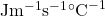Explanation:

The thermal conductivity of an object is defined as the measure or the ability of the object to transfer heat or conduct heat through its body.

In the context, the thermal conductivity of the material is given as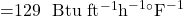And it is given that :

1 Btu = 1055 J

1 ft = 0.3048 m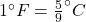We know that 1 h = 3600 s

So the thermal conductivity of the material inis  :

Thermal conductivity :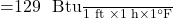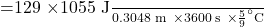=  223.25